## Number-Systems

 Question 1
If a variable can take only integral values from 0 to n, where n is an integer, then the variable can be represented as a bit field whose width is (the log in the Solutions are to the base 2, and [log n] means the floor of log n)
 A [log(n)] + 1 bits B [log (n-1)) + 1 bits C [log (n+1)] + 1 bits D None of the above
Digital-Logic-Design       Number-Systems       ISRO-2018
Question 1 Explanation:Question 2
Given √(224)r = 13r the value of radix r is
 A 10 B 8 C 6 D 5
Digital-Logic-Design       Number-Systems       ISRO-2018
Question 2 Explanation:
√(224)r = 13r
For f(x) to be maximum
f'(x) = 4x - 2 = 0
⇒ x = 1/2
So at x = 1/2, f(x) is an extremum (either maximum or minimum).
f(2) = 2(2)2 - 2(2) + 6 = 10
f(1/2) = 2 × (1/2)2 - 2 × 1/2 + 6 = 5.5
f(0) = 6
So, the maximum value is at x=2 which is 10 as there are no other extremum for the given function.
 Question 3
When two n-bit binary numbers are added the sum will contain at the most
 A n bits B (n+3) bits C (n+2) bits D (n+1) bits
Digital-Logic-Design       Number-Systems       ISRO-2017 May
Question 3 Explanation:
→ When two n-bit binary numbers are added the sum will contain at the most (n+1) bits
Example = 2 Decimal numbers are (7)10 and (7)10
= Equivalent binary numbers are (111)2 + (111)2
= Adding two binary numbers, the final result will be n+1 number (1110)2
 Question 4
(1217)8 is equivalent to
 A (1217)16 B (028F)16 C (2297)1o D (0B17)16
Digital-Logic-Design       Number-Systems       ISRO-2017 May
Question 4 Explanation:
(1217)8=(001 010 001 111)2
=(0010 1000 1111)2
=(2 8 F)16
 Question 5
The logic circuit given below converts a binary code y1,y2,y3 intoA Excess-3 code B Gray code C BCD code D Hamming Code
Digital-Logic-Design       Number-Systems       ISRO-2016
Question 5 Explanation:
X1= Y1
X2= Y1⊕ Y2
X3= Y2 ⊕ Y3
 Question 6
If 12A7C16 = X8, then the value of X is
 A 224174 B 425174 C 6173 D 225174
Digital-Logic-Design       Number-Systems       ISRO-2016
Question 6 Explanation:
Given, (12A7C)16 = (0001 0010 1010 0111 1100)2
MAke blocks of 3 bits each from LSB to MSB.
(Note: In the last block append zeros (as MSBs) if number bits is not three)
(000 010 010 101 001 111 100)
Each of the above blocks represents a digit in base 8 and they can be converted to base 8 as shown below.
= (0 2 2 5 1 7 4)8
 Question 7
The Excess-3 code is also called
 A Cyclic Redundancy Code B Weighted Code C Self-Complementing Code D Algebraic Code
Digital-Logic-Design       Number-Systems       ISRO-2016
Question 7 Explanation:
Excess-3 code is also called Self-Complementing Code. Because 1’s complement of an excess-3 number is equivalent to 9’s complement of the corresponding decimal digit.
→ In excess-3 code, each of the 4-bit numbers represents decimal digit which is 3 less than the actual decimal digit. So the bits have no fixed weight.
Excess-3 code is neither CRC nor Algebraic Code which is used for error detection and/or correction.
 Question 8
Which of the following binary number is the same as its 2’s complement?
 A 1010 B 0101 C 1000 D 1001
Digital-Logic-Design       Number-Systems       ISRO-2016
Question 8 Explanation:Hint: Number of bits=4
(Decimal value of maximum 4-bit number +1 )/2= (15+1)/2=8
 Question 9
The addition of 4-bit, two’s complement, binary numbers 1101 and 0100 results in
 A 0001 and an overflow B 1001 and no overflow C 0001 and no overflow D 1001 and an overflow
Digital-Logic-Design       Number-Systems       ISRO CS 2009
Question 9 Explanation:
Number one is 0100 (4-Decimal value)
Another number is 1101(-3 is decimal value)
Adding of -3 and 4, the result is 1 and there is no overflow
 Question 10
What is the decimal value of the floating-point number C1D00000 (hexadecimal notation)? (Assume 32-bit, single precision floating point IEEE representation)
 A 28 B -15 C -26 D -28
Digital-Logic-Design       Number-Systems       ISRO CS 2011
Question 10 Explanation:
Floating Point number in Hexadecimal = C1D00000
Floating Point number in Binary = 1100 0001 1101 0000 0000 0000 0000 0000
In 32-bit, single precision floating point IEEE representation, first MSB represents sign of mantissa: 1 is used to represent a negative mantissa and 0 for a positive value of mantissa, next 8 bits are for exponent value and then 23 bits represents mantissa.
Value of exponent = 131-127 = 4
Mantissa = -1.1010000 0000 0000 0000 0000
Floating point number = -1.1010000 0000 0000 0000 0000
Converting the above one into decimal no -(1*20+1*2-1*0*2-2+1*2-2+0* 2-3 +.....)
= -(1+½+⅛)=-13/8
Decimal value =sign*Exponent*mantissa=1*4*-13/8

= -26
 Question 11
In the standard IEEE 754 single precision floating point representation, there is 1 bit for sign, 23 bits for fraction and 8 bits for exponent. What is the precision in terms of the number of decimal digits?
 A 5 B 6 C 7 D 8
Digital-Logic-Design       Number-Systems       ISRO CS 2014
Question 11 Explanation:
A floating-point variable can represent a wider range of numbers than a fixed-point variable of the same bit width at the cost of precision. A signed 32-bit integer variable has a maximum value of 231 − 1 = 2,147,483,647, whereas an IEEE 754 32-bit base-2 floating-point variable has a maximum value of (2 − 2−23) × 2127 ≈ 3.402823 × 1038.
In the IEEE 754-2008 standard, the 32-bit base-2 format is officially referred to as binary32; it was called single in IEEE 754-1985. IEEE 754 specifies additional floating-point types, such as 64-bit base-2 double precision and, more recently, base-10 representations.
We can convert the binary into decimal representation by using the following steps
let the number of digits in decimal digits be ‘x’
2-23 = 10-x
After taking log on both sides
log210-x =log2 2-23
-x log210 = -23log22 (The value of log22=1)
-3.322 x = -23 (The value of log210 = 3.321928)
x = 6.92
 Question 12
How many different BCD numbers can be stored in 12 switches? (Assume two position or on-off switches)
 A 212 B 212-1 C 1012 D 103
Digital-Logic-Design       Number-Systems       ISRO CS 2014
Question 12 Explanation:
Step-1: A binary-coded decimal (BCD) is a class of binary encodings of decimal numbers where each decimal digit is represented by a fixed number of bits, usually four (or) eight.
Step-2: Decimal number 0 can be represented 0000 and 9 can be represented by using 1001.
Step-3: A switch can store maximum 1 bit data that may be either 0 (or) 1. In switch terminology, 0 means “off” and 1 means “on”. With 4 bit we can represent 10 BCD numbers.
Step-4: A BCD digit can be from 0 to 9 (total 10 possibility).
Step-5: Different possible BCD numbers in 12 switches are = 10*10*10
= 1000
= 103
 Question 13
The range of the numbers which can be stored in an eight bit register is
 A -128 to +127 B -128 to +128 C -999999 + +999999 D none of these
Digital-Logic-Design       Number-Systems       Nielit Scientist-C 2016 march
Question 13 Explanation:
There are 2​ 8​ (256) different possible values for 8 bits. When unsigned, it has possible values ranging from 0 to 255; when signed, it has -128 to 127.
 Question 14
The excess 3 code is also called
 A Cyclomatic redundancy code B Weighted code C Self complementing code D algebraic code
Digital-Logic-Design       Number-Systems       Nielit Scientist-C 2016 march
Question 14 Explanation:
Excess-3 is a self-complementing code. This is because in Excess-3 code we get the 9's complement of a number by just complementing each bit that means by replacing a '0' by '1' and '1' by '0'
 Question 15

In computers, subtraction is generally carried out by

 A 1’s complement B 10’s complement C 2’s complement D 9’s complement
Digital-Logic-Design       Number-Systems       UGC-NET DEC Paper-2
Question 15 Explanation:
• In computers, subtraction is generally carried out by 2’s complement.
• In two's-complement representation, positive numbers are simply represented as themselves, and negative numbers are represented by the two's complement of their absolute value.
• In the subtraction there may possibility of negative number as a result.
 Question 16
A decimal number has 30 digits. Approximately, how many digits would the binary representation have?
 A 30 B 60 C 90 D 120
Digital-Logic-Design       Number-Systems       Nielit Scientist-B CS 2016 march
Question 16 Explanation:
Here, 30 digits numbers means 123....30. 10​30​ -1=1000000000000000000000000000000-1=999999999999999999999999999999
Therefore, it takes approximately above 90 binary numbers. So, 120 is correct answer.
 Question 17
The result of the subtraction FD​16 - ​8816​ is
 A 75​ 16 B 65 16 C 5E 16 D 10 16
Digital-Logic-Design       Number-Systems       Nielit Scientist-B CS 2016 march
Question 17 Explanation:
Step-1: Convert Hexadecimal numbers into decimal numbers.
(FD)​ 16<​/sub> = (253)​ 10
(88)​ 16<​/sub> = (136)​ 10
Step-2: Perform subtraction 253-136=(117)​ 10
Step-3: Convert (117)​ 10 ​ =(75)​ 16
 Question 18
What will be the Excess-3 code for 1001?
 A 1001 B 1010 C 1011 D 1100
Digital-Logic-Design       Number-Systems       Nielit Scientist-B CS 4-12-2016
Question 18 Explanation:
Excess-3 number starts with 3. Here, 1001 menas decimal number-9. So, we have to add +3.
12 equivalent binary number is 1100.
 Question 19
The Decimal equivalent of the Hexadecimal number (A09D)16 is
 A 31845 B 41117 C 41052 D 32546
Digital-Logic-Design       Number-Systems       Nielit Scientist-B CS 4-12-2016
Question 19 Explanation:
Given Hexadecimal number is ​ (A09D)16
A decimal number is the sum of the digits multiplied with its power of 10.
(A09D)16​ is equal to each digit multiplied with its corresponding power of 16:
Ax16​ 3​ +0x16​ 2​ +9x16​ 1​ +Dx16​ 0​ =(10x4096+144+13x1) [ Where A=10,D=13]
=40960+144+13=41117
 Question 20
Which of the given number has its IEEE-754 32 bit floating point representation as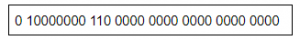A 2.5 B 3 C 3.5 D 4.5
Digital-Logic-Design       Number-Systems       ISRO CS 2015
Question 20 Explanation:
→ Sign bit S = 0 (It means positive number)
→ E=1000 0000B = 128D (in normalized form)
→ Fraction is 1.11B (with an implicit leading 1) = 1 + 1×2-1 + 1×2-2
= 1.75D
→ The number is +1.75 × 2(128-127)
= +3.5D
 Question 21
The range of integers that can be represented by an n bit 2’s complement number system is:
 A – 2n – 1 to 2n – 1 – 1 B – (2n – 1 – 1) to (2n – 1 – 1) C – 2n – 1 to 2n – 1 D – (2n – 1 + 1) to (2n – 1 – 1)
Digital-Logic-Design       Number-Systems       ISRO CS 2015
Question 21 Explanation:
In 2’s complement numbers, the range of integers are from -2n-1 to 2n-1 – 1
 Question 22
The code which uses 7 bits to represent a character is
 A ASCII B BCD C EBCDIC D Gray
Digital-Logic-Design       Number-Systems       ISRO CS 2015
Question 22 Explanation:
→ ISO/IEC 646, like ASCII, is a 7-bit character set. It does not make any additional codes available, so the same code points encoded different characters in different countries. Escape codes were defined to indicate which national variant applied to a piece of text, but they were rarely used, so it was often impossible to know what variant to work with and, therefore, which character a code represented, and in general, text-processing systems could cope with only one variant anyway.
→ Extended Binary Coded Decimal Interchange Code (EBCDIC) is an 8-bit binary code for numeric and alphanumeric characters.
→ BCD encoding uses 4 bits to represent each digit from the range 0 to 9 in its binary form.
→ In case of Gray codes, any number of bits can be used to represent a character, according to the requirement.
 Question 23
The number of 1’s in the binary representation of (3*4096 + 15*256 + 5*16 + 3) are:
 A 8 B 9 C 10 D 12
Digital-Logic-Design       Number-Systems       ISRO CS 2015
Question 23 Explanation:
Binary expression of (3*4096 + 15*256 + 5*16 + 3)
=(12,288+3840+80+3)
=(16211)10
=‭(0011111101010011‬)2
Total number of 1’s in binary representation is 10.
 Question 24
The decimal number has 64 digits.The number of bits needed for its equivalent binary representation is?
 A 200 B 213 C 246 D 277
Digital-Logic-Design       Number-Systems       ISRO CS 2015
Question 24 Explanation:
Consider three digits(1,2,3) of decimal numbers.Maximum number, we can generate by that three digits are 103-1 which is 999.
Then, Decimal number has 64 digits, so maximum number is 1064-1
Similarly, in the binary representation with “n” bits the maximum number is 2n-1
So we can write 1064 –1 = 2n – 1 --->1064 = 2n
After taking log2 on both sides
log22n=log21064
n log22=64 log 210
n=64*(3.322) [ log22=1 & log210 =3.322]
n=212.608
n=213
 Question 25

Suppose x and y are floating point variables that have been assigned the values x = 8.8 and y = 3.5. What will be the value of the following arithmetic expression?

2 * x / 3 * y

 A 20.3333 B 24.4545 C 16.3535 D 20.5333
Digital-Logic-Design       Number-Systems       JT(IT) 2018 PART-B Computer Science
Question 25 Explanation:
x = 8.8 y=3.5
= 1 (equal priority
Associativity (left to Right)
(((2 * x)/3) * y)
((2 x *) / 3) * y)
(2x * 31) * y
2x * 31) y*
(2x * 31 y*
Put the 2 * 8.8 * 3 / 3.5 *
= 20.53333
 Question 26
A decimal has 25 digits. the number of bits needed for its equivalent binary representation is approximately
 A 50 B 74 C 40 D 60 E None of these
Digital-Logic-Design       Number-Systems       Nielit Scientific Assistance IT 15-10-2017
Question 26 Explanation:
Consider three digits(1,2,3) of decimal numbers.Maximum number, we can generate by that three digits are 10​ 3​ -1 which is 999.
Then, Decimal number has 25 digits, so maximum number is 10​ 25​ -1
Similarly, in the binary representation with “n” bits the maximum number is 2​n​ -1
So we can write 10​ 25​ –1 = 2​ n​ – 1 --->10​ 25​ = 2​ n After taking log​ 2​ on both sides
log​ 2​ 2​ n​ =log ​ 2​ 10​ 25
n log​ 2​ 2=25 log ​ 2​ 10
n=25*(3.322) [ log​ 2​ 2=1 & log​ 2​ 10 =3.322]
n=83
 Question 27

What is the base(radix) of the number system whose numbers 312, 20 and 13.1 satisfy the following equation?

312/20 = 13.1
 A 8 B 4 C 5 D 6
Digital-Logic-Design       Number-Systems       JT(IT) 2018 PART-B Computer Science
Question 27 Explanation:
Let base of the number system is r.
(3r2 + r + 2) / 2r = (r + 3 + 1/r)
(3r2 + r + 2) / 2r = (r2 + 3r + 1) / r
(3r2 + r + 2) = (2r2 + 6r + 2)
r2 - 5r = 0
Therefore, r = 5
 Question 28

What is the hexadecimal representation of the decimal number 8537?

 A (2059)16 B (2159)16 C (2195)16 D (2157)16
Digital-Logic-Design       Number-Systems       JT(IT) 2018 PART-B Computer Science
Question 28 Explanation:
Step-1: First convert decimal number into binary number
Step-2: (8537)10 = (0010000101011001)2
Step-3: Divide binary number into 4 segments (0010 0001 0101 1001)2
Step-4: Write equivalent number of hexadecimal (2159)16
 Question 29
Shifting a register content to left by one bit is equivalent to____
 A Division by 2 B Addition by 2 C Multiplication by 2 D Subtraction by 2
Digital-Logic-Design       Number-Systems       KVS 22-12-2018 Part-B
Question 29 Explanation:
→ The left-shift operator (<<), which moves the bits of shift-expression to the left.
→ The bit positions that have been vacated by the shift operation are zero-filled.
→ For example a=5 and equivalent binary value is 101 and shifting one bit left side means the result binary value is 1010 whose decimal value is 10
 Question 30
What shall be the 2’s complement represented of -24 in a 16 bit computer?
 A 1111 1111 1110 1011 B 1111 1111 1110 1001 C 1111 1111 1110 0111 D 1111 1111 1110 1000
Digital-Logic-Design       Number-Systems       KVS 22-12-2018 Part-B
Question 30 Explanation:
Negative numbers are represented in 2’s complement form.
The binary equivalent of 24 is 0000 0000 0001 1000
One’s complement is 1111 1111 1110 0111 (Flipping the bits 1 by 0 and 0 by 1)
Two’s complement is 1111 1111 1110 1000 (adding 1 to the LSB bit)
 Question 31
A decimal has 25 digits. the number of bits needed for its equivalent binary representation is approximately
 A 50 B 74 C 40 D None of the above
Digital-Logic-Design       Number-Systems       Nielit Scientific Assistance CS 15-10-2017
Question 31 Explanation:
Consider three digits(1,2,3) of decimal numbers.Maximum number, we can generate by that three digits are 10​ 3​ -1 which is 999.
Then, Decimal number has 25 digits, so maximum number is 10​ 25​ -1
Similarly, in the binary representation with “n” bits the maximum number is 2​ 25​ -1
So we can write 10​ 25​ –1 = 2​ n​ – 1 → 10​ 25​ = 2​ n
After taking log​ 2​ on both sides
log​ 2​ 2​ n​ =log ​ 2​ 10​ 25
n log​ 2​ 2=25 log ​ 2​ 10
n = 25 log ​ 2​ 10
n = 25 x 3.3 [ log​ 2​ 2=1 & log​ 2​ 10 =3.322]
n = 82.5
Note: Original question paper given option D is 60. But actual answer is 82.5.
 Question 32
Which of the following is minimum error code?
 A Octal code B Binary Code C Gray code D Excess-3 Code
Digital-Logic-Design       Number-Systems       Nielit Scientific Assistance CS 15-10-2017
Question 32 Explanation:
→ "Gray code" as an alternative name is "reflected binary code". one of those also lists "minimum error code" and "cyclic permutation code" among the names.
→ Gray codes are widely used to facilitate error correction in digital communications such as digital terrestrial television and some cable TV systems.
 Question 33
In single-precision, double-precision and extended-precision representation of floating point numbers, as defined by ANSI/IEEE standard 754-1985, the no.of bits used are____ respectively.
 A 32,64 and 80 B 32,64 and 128 C 16,32 and 64 D 16,32 and 80
Digital-Logic-Design       Number-Systems       KVS 22-12-2018 Part-B
Question 33 Explanation:Extended precision, the third format, is usually an 80-bit word, with 1 bit sign, 15 bit exponent and 64 bit significand, with leading bit of a normalized number not hidden
 Question 34
If the original size of data is 40 then after adding error detection redundancy bit the size of data length is
 A 26 B 36 C 46 D 56
Digital-Logic-Design       Number-Systems       Nielit Scientific Assistance CS 15-10-2017
Question 34 Explanation:
→ Imagine that we want to design a code with m message bits and r check bits that will allow all single errors to be corrected.
→ Each of the 2​ m​ legal messages has n illegal codewords at a distance of 11 from it.
→ These are formed by systematically inverting each of the n bits in the n-bit codeword formed from it. Thus, each of the 2​ m​ legal messages requires n+1 bit patterns dedicated to it.
→ Since the total number of bit patterns is 2​ n​ ,​ We must have (n+1)2​ m​ ≤ 2​ n​ .
→ Using n=m+r, this requirement becomes
= (m+r+1) ≤ 2​ r
= 40+6+1 ≤ 26
= 47 ≤ 2
r=6
Message size will be 6+40=46
 Question 35

Which of the following is a recursive algorithm to convert a positive decimal integers into equivalent binary integers?

 AB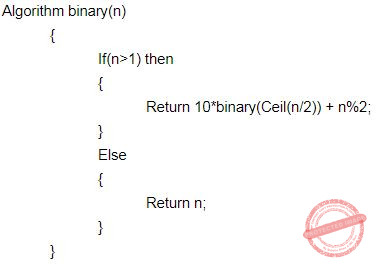C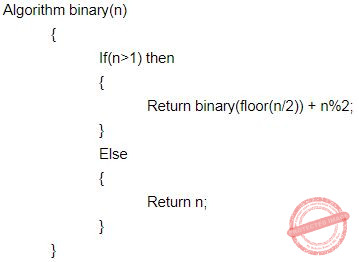D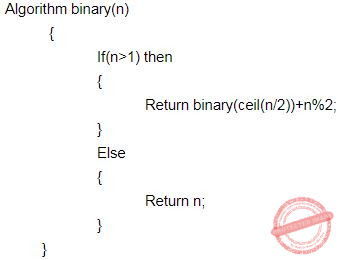Digital-Logic-Design       Number-Systems       JT(IT) 2018 PART-B Computer Science
Question 35 Explanation:
Algorithm:
Take decimal number is 12.
Step-1 → 12%2 which is equal to 0+10*(⌊12/2⌋)%2
Step-2 → 6%2 which is equal to 0+10*(⌊6/2⌋)%2
Step-3 → 3%2 which is equal to 1+10*(⌊3/2⌋)%2
Step-4 → 1%2 which is equal to 1+10*(⌊1/2⌋)%2
 Question 36
Consider an arbitrary number system with the independent digits as 0,1 and X. What is the radix of this number system?
 A 1 B 2 C 3 D 4
Digital-Logic-Design       Number-Systems       KVS DEC-2013
Question 36 Explanation:
→ The radix of a number system is the number of unique digits, including, zero, that are used to represent larger numbers. In the decimal system that would be 0 to 9.
→ In the question, the unique digits are 0,1 and X(possible x value is 2) then number system is 3;
 Question 37
The hamming(7,4) code for 0000 using even parity is
 A 0000000 B 1111111 C 2222222 D 12121212
Digital-Logic-Design       Number-Systems       KVS DEC-2013
Question 37 Explanation:
● Hamming(7,4) is a linear error-correcting code that encodes four bits of data into seven bits by adding three parity bits.
● The data is ​ 0000 and Hamming(7,4) transmitted is 0000000
 Question 38
The number of bits required to represent decimal number 4096 in binary form is___
 A 16 B 10 C 12 D 13
Digital-Logic-Design       Number-Systems       KVS DEC-2017
Question 38 Explanation:
(4098)​ 10​ =(1000000000010)​ 2
So, total 13 bits required to represent 4096 decimal number.
 Question 39

The signed 2’s complement representation of -33 is:

 A 11011111 B 00100001 C 01011111 D 10100001
Digital-Logic-Design       Number-Systems       JT(IT) 2016 PART-B Computer Science
Question 39 Explanation:
Step-1: -33 corresponding positive number 00100001
Step-2: Perform 2’s complement.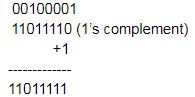Question 40

Using signed 2’s complement subtraction the result of 11111010-11110011 is:

 A 10000111 B 00000111 C 10001101 D 00001101
Digital-Logic-Design       Number-Systems       JT(IT) 2016 PART-B Computer Science
Question 40 Explanation:
11111010 → 250(Decimal)
-11110011 → -243(Decimal)
Step1: convert signed number into 2’s complement
11110011
00001100 → (1’s complement)
+1
-------------
00001101 → (2’s complement)
Step 2: Add 11111010 into 2’s complement number
11111010
00001101
--------------
00000111 → solution
 Question 41
How many bits are used in the exponent part of IEEE single precision for the representation of floating point numbers?
 A 32 bits B 8 bits C 16 bits D 24 bits
Digital-Logic-Design       Number-Systems       KVS DEC-2017
Question 41 Explanation:
The IEEE 754 standard specifies a binary floating point format(binary32) as having:
→ Sign bit: 1 bit
→ Exponent width: 8 bits
→ Significand precision: 24 bits (23 explicitly stored)
 Question 42
Convert the following octal number into its decimal equivalent: 2 3 7 4 in octal
 A (10208)10 B (1276)10 C (2374)10 D (1272)10
Digital-Logic-Design       Number-Systems       KVS 30-12-2018 Part B
Question 42 Explanation:
(2374)8=2x83+3x82+7x81+4x80=1024+192+56+4=1276
 Question 43
Represent the decimal number 3.248*104 into a single precision floating point binary number(using standard format).
 A 0|10001101|11111011100000000000000 B 0|11001101|11111011100000000000000 C 1|11001101|11111011100000000000000 D 0|10001110|11111011100000000000000
Digital-Logic-Design       Number-Systems       KVS 30-12-2018 Part B
Question 43 Explanation:
Given number is in base 10. Convert it to base-10.
3.248x104 =32480= 1111110111
= 1.111110111 x 214
Mantissa = 11111011100...00
Biased exponent = 14 +127= 141 = 10001101
 Question 44
What is the octal equivalent of the hexadecimal number 132A?
 A 46252 B 11450 C 11452 D 45250
Digital-Logic-Design       Number-Systems       KVS DEC-2017
Question 44 Explanation:
Step-1: Convert hexadecimal number in binary format. It is nothing but representing 4 binary values of each character.
Step-2: (132A)​ 16​ =(0001 0011 0010 1010)​ 2 Step-3: Divide 4 binary numbers in to 3 binary numbers from right to left.
Step-4: (0 001 001 100 101 010)​ 2
(0 1 1 4 5 2)​ 8
 Question 45
The Octal equivalent of the binary number 1011101011 is :
 A 7353 B 1353 C 5651 D 5657
Digital-Logic-Design       Number-Systems       UGC NET CS 2017 Nov- paper-2
Question 45 Explanation:
We have to divide binary number into 3 bit pairs from LSB.
1 011 101 011
1 3 5 3
(1011101011)​ 2​ = (1353)​ 8
 Question 46
Which is the hexadecimal number equivalent to the octal number 46250
 A 4AC8 B 4CA8 C CCA8 D 4CA4
Digital-Logic-Design       Number-Systems       KVS 30-12-2018 Part B
Question 46 Explanation:
Given octal number is 46250 and the corresponding binary number we will get by writing each decimal digit into three binary digits form which is 100110010101000
Now group the four digits from LSB and write corresponding equivalent of Hexadecimal digit of binary digits.
The Hexadecimal number of 100(4) 1100(C) 1010(A) 1000(8) which is nothing but 4CA8
 Question 47
Let m=(313)​ 4​ and n=(322)​ 4​ . Find the base 4 expansion of m+n.
 A (635)​ 4 B (32312)​ 4 C (21323)​ 4 D (1301)​ 4
Digital-Logic-Design       Number-Systems       UGC NET CS 2017 Nov- paper-2
Question 47 Explanation:
In this problem, they are asking to find (m+n)​ 4
→ We are using addition for decimal number system. But in this problem m and n values are base 4. So, we can’t add directly.
Step-1: We have to perform base 4 into decimal values of m and n.
m = 3*42 + 1*41 + 3*40
m = 48 + 4 + 3
m = 55
n= 3*42 + 2*41 +2*40
n= 48 + 8 +2
n= 58
Step-2: The resultant decimal values should perform addition.
m+n = 55 + 58
m+n = 113
Step-3: Finally we have to convert decimal value into base 4 value.(1301)4
 Question 48
Convert the octal number 0.4051 into its equivalent decimal number.
 A 0.51001 B 0.2096 C 0.52 D 0.4192
Digital-Logic-Design       Number-Systems       UGC NET CS 2017 Jan -paper-2
Question 48 Explanation:Question 49
The hexadecimal equivalent of the octal number 2357 is :
 A 2EE B 2FF C 4EF D 4FE
Digital-Logic-Design       Number-Systems       UGC NET CS 2017 Jan -paper-2
Question 49 Explanation:
Step-1: Convert octal number into binary number
(2357)​ 8​ = (010 011 101 111)​ 2
Step-2: Divide 4 bits from LSB then will get hexadecimal number
0100 1110 1111
2 E F
(2EF)​ 16​ = (2357)​ 8
 Question 50
If X is a binary number which is power of 2, then the value of X & (X – 1) is :
 A 11....11 B 00.....00 C 100.....0 D 000......1
Digital-Logic-Design       Number-Systems       UGC NET CS 2017 Jan -paper-2
Question 50 Explanation:
Given data,
→ X is binary number which is power of 2. It means, we have to take powers of 2 numbers only.
Ex: 1,2,4,8,16,32,..,
Let X=4
X=4 equivalent binary number is 100
X-1=3 equivalent binary number is 011
100
011
-----
000 (AND operation)
-----
Ex-2:
X=8 and X-1=7
8 binary value is
1000
7 binary number is 0111
--------
0000(AND operation)
--------
So, Option B is correct answer.
 Question 51
The octal number 326.4 is equivalent to
 A (214.2)​ 10​ and (D6.8))​ 16 B (212.5)​ 10​ ​ and (D6.8))​ ​ 16 C (214.5)​ 10​ and (D6.8))​ ​ 16 D (214.5)​ 10​ and (D6.4))​ ​ 16
Digital-Logic-Design       Number-Systems       UGC NET CS 2016 Aug- paper-2
Question 51 Explanation:
(326.4) 8 = ( ?)
Step1: First convert given octal no. to binary number because it will be easier to solve this way.Step 2: Now convert above binary no. into decimal .
(011010110.100) 2
= ( 1 * 2 7 ) + ( 1 × 2 6 ) + ( 1 × 2 4 ) + ( 1 × 2 2 ) + ( 1 × 2 1 ) · [ 1 × ( 1/2) ]
= (214.5) 10
(326.4) 8 = (?) 16 Step 1: Convert given octal no. into binary no.Step 2: Now convert above binary no. into decimal .
(011010110.100) 2
= ( 1 * 2 7 ) + ( 1 × 2 6 ) + ( 1 × 2 4 ) + ( 1 × 2 2 ) + ( 1 × 2 1 ) · [ 1 × ( 1/2) ]
= (214.5) 10
(326.4) 8 = (?) 16Question 52
Which of the following is the most efficient to perform arithmetic operations on the numbers?
 A Sign-magnitude B 1’s complement C 2’s complement D 9’s complement
Digital-Logic-Design       Number-Systems       UGC NET CS 2016 Aug- paper-2
Question 52 Explanation:
2’s complement has single representation for zero , but Sign-magnitude, 1’s complement and 9’s complement have two representations for 0 (i.e., both positive zero and negative zero). While doing arithmetic operations like addition or subtraction using 1's complement(or 9's complement), we have to add an extra carry bit, i.e 1 to the result to get the correct answer. 2's complement doesn't require such extra calculation.
 Question 53
An example of a binary number which is equal to its 2​ ’s complement is :
 A 1100 B 1001 C 1000 D 1111
Digital-Logic-Design       Number-Systems       UGC NET CS 2004 Dec-Paper-2
Question 53 Explanation:
Option-A: 1100 convert into 2’s complement is
1100
1’s complement: 0011
2’s complement: 1
--------
0100
Option-B: 1001 convert into 2’s complement is
1001
1’s complement: 0110
2’s complement:
1
--------
0111
---------
Option-C: 1000 convert into 2’s complement is
1000
1’s complement: 0111
2’s complement:
1
--------
1000
---------
Option-D: 1111 convert into 2’s complement is
1111
1’s complement: 0000
2’s complement:
1
--------
0001
---------
 Question 54
Which of the following is divisible by 4 ?
 A 100101100 B 1110001110001 C 11110011 D 10101010101010
Digital-Logic-Design       Number-Systems       UGC NET CS 2005 Dec-Paper-2
Question 54 Explanation:
Option-A: (100101100)​ 2​ = (300)​ 10
300 is divisible by 4
Option-B: (1110001110001)​ 2​ = (7281)​ 10
7281 is not divisible by 4
Option-C: (11110011)​ 2​ = (243)​ 10
243 is not divisible by 4
Option-D: (10101010101010)​ 2​ = (10,922)​ 10
10,922 is not divisible by 4.
 Question 55
(101011)​ 2​ =(53)​ b​ , then ‘b’ is equal to :
 A 4 B 8 C 10 D 16
Digital-Logic-Design       Number-Systems       UGC NET CS 2005 june-paper-2
Question 55 Explanation:
We are dividing binary number into 3 then
101 011
5 3
(101011)​ 2 = (53)​ 8
Suppose, we are dividing binary digits into 4 then
0010 1011
2 B
So, it is not hexadecimal number.
 Question 56
Which of the following binary number is the same as its 2’s complement :
 A 1010 B 0101 C 1000 D 1001
Digital-Logic-Design       Number-Systems       UGC NET CS 2005 june-paper-2
Question 56 Explanation:
Option-A: 1010 convert into 2’s complement is
1010
1’s complement: 0101
2’s complement: 1
--------
0110
--------- Option-B: 0101 convert into 2’s complement is
0101
1’s complement: 1010
2’s complement: 1
--------
1011
---------
Option-C: 1000 convert into 2’s complement is
1000
1’s complement: 0111
2’s complement: 1
--------
1000
---------
Option-D: 1001 convert into 2’s complement is
1001
1’s complement: 0110
2’s complement: 1
--------
0111
---------
 Question 57
The hexadecimal equivalent of (10111)​ 2​ ×(1110)​ 2 is :
 A 150 B 241 C 142 D 101011110
Digital-Logic-Design       Number-Systems       UGC NET CS 2006 Dec-paper-2
Question 57 Explanation:
Step-1: First convert binary number into decimal number
(10111)​ 2​ = (23)​ 10
(1110)​ 2​ = (14)​ 10
Step-2: Perform multiplication 23*14=(322)​ 10
Step-3: Convert (322)​ 10​ into hexadecimal number (322)​ 10​ = (142)​ 16
 Question 58
An example of a self complementing code is :
 A 8421 code B Gray code C Excess-3 code D 7421 code
Digital-Logic-Design       Number-Systems       UGC NET CS 2006 Dec-paper-2
Question 58 Explanation:
→ Excess-3 code is also called Self-Complementing Code. Because 1’s complement of excess-3 number is equivalent to 9’s complement of corresponding decimal digit.
→ In excess-3 code, each of the 4-bit number represents decimal digit which is 3 less than actual decimal digit. So the bits have no fixed weight.
→ Excess-3 code is ​ neither​ CRC ​ nor​ Algebraic Code which are used for error detection and/or correction.
 Question 59
The number of 1​ ’s present in the binary representation of (3*512 + 7*64 +5*8 +3)​ 10​ is :
 A 8 B 9 C 10 D 11
Digital-Logic-Design       Number-Systems       UGC NET CS 2006 June-Paper-2
Question 59 Explanation:
(3*512 + 7*64 +5*8 +3) =2027
(2027)​ 10​ = (111 1110 1011)​ 2
Here, total number of 1’s are 9.
 Question 60
​ In a weighted code with weight 6, 4, 2, -3 the decimal 5 is represented by :
 A 0101 B 0111 C 1011 D 1000
Digital-Logic-Design       Number-Systems       UGC NET CS 2006 June-Paper-2
Question 60 Explanation:
The decimal value 5 is represented by 1011.
= 6*1 + 4*0 + 2*1 + -3*1
= 6+2-3
=5
 Question 61
Digital-Logic-Design       Number-Systems       UGC NET CS 2014 Dec-Paper-2
Question 61 Explanation:
→ Each digit is represented by a 4-bit BCD code.
→ To add two 4-bit number, we need 1 Half Adder(to add LSBs) and 3 Full Adders(remaining three bits of both number along with carry bits).
→ To make the resultant Sum as valid BCD sum, we need to add 0110 to the sum.
→ This can be done with 1 Half adder and 2 Full Adder
(Note: LSB bit of 0110 is always zero. So there is no need of ADDER to add LSBs.)
 Question 62
The Excess-3 decimal code is a self-complementing code because
 A The binary sum of a code and its 9’s complement is equal to 9. B It is a weighted code C Complement can be generated by inverting each bit pattern D The binary sum of a code and its 10’s complement is equal to 9
Digital-Logic-Design       Number-Systems       UGC NET CS 2014 Dec-Paper-2
Question 62 Explanation:
The Excess-3 decimal code is a self-complementing code because complement can be generated by inverting each bit pattern.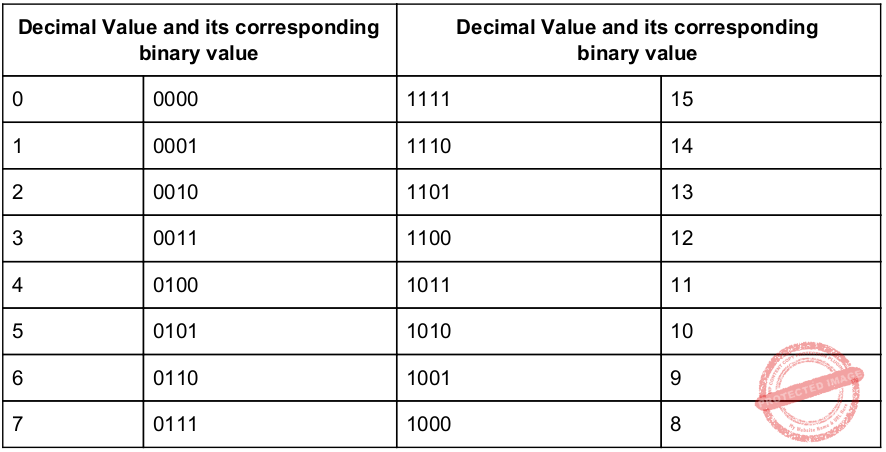Question 63
The range of representable normalized numbers in the floating point binary fractional representation in a 32-bit word with 1-bit sign, 8-bit excess 128 biased exponent and 23-bit mantissa is
 A 2​ -128​ to (1 – 2​ –23​ ) * 2​ 127 B (1 – 2​ –23​ ) * 2​ ​ -127 ​ to 2​ 128 C (1 – 2​ –23​ ) * 2​ –127​ to 2​ 23> D 2​ –129​ to (1 – 2​ –23​ ) * 2​ 127
Digital-Logic-Design       Number-Systems       UGC NET CS 2014 Dec-Paper-2
Question 63 Explanation:
The range of representable normalized numbers in the floating point binary fractional representation in a 32-bit word with 1-bit sign, 8-bit excess 128 biased exponent and 23-bit mantissa is​ ​ 2 –129 to (1 – 2​ –23​ ) * 2​ 127
 Question 64
The decimal floating point number -40.1 represented using IEEE-754 32-bit representation and written in hexadecimal form is
 A 0xC2206000 B 0xC2006666 C 0xC2006000 D 0xC2206666
Digital-Logic-Design       Number-Systems       UGC NET CS 2018-DEC Paper-2
Question 64 Explanation:
1. Fraction part can be converted into binary form by multiplying it with 2 and taking non fractional part of the product. Take fractional part and multiply again as explained above.
0.1 x 2= 0.2 → 0
0.2 x 2= 0.4 → 0
0.4 x 2= 0.8 → 0
0.8 x 2= 1.6 → 1
0.6 x 2= 1.2 → 1
(0.1)​ 10​ = (0.00011)​ 2
(40)​ 10​ = (101000)​ 2
101000.00011
Normalize the number
1.0100000011 x 2​ 5
Biased exponent= 5+127= 132=(1000 0100)​ 2
Mantissa= 01000000110000000000000
Sign= 1Question 65
The IEEE single-precision and double-precision format to represent floating-point numbers, has a length of ______ and ______ respectively.
 A 8 bits and 16 bits B 16 bits and 32 bits C 32 bits and 64 bits D 64 bits and 128 bits
Digital-Logic-Design       Number-Systems       UGC NET CS 2013 Sep-paper-2
Question 65 Explanation:
The IEEE single-precision and double-precision format to represent floating-point numbers, has a length of 32 bits and 64 bits respectively.
 Question 66
Given that (292)10 = (1204)x in some number system x. The base x of that number system is
 A 2 B 8 C 10 D None of the above
Digital-Logic-Design       Number-Systems       UGC NET CS 2013 Dec-paper-2
Question 66 Explanation:
Option-A: It is false because it is equivalent to (292)10 =(100100100)2 Option-B: It is false because it is equivalent to (292)10 =(444)8 Option-B: It is false because it is equivalent to (292)10 =(292)10 So, option-D is the correct answer. The actual x value is 6.
 Question 67
If an integer needs two bytes of storage, then the maximum value of a signed integer is
 A 216 – 1 B 215 – 1 C 216 D 215
Digital-Logic-Design       Number-Systems       UGC NET CS 2012 June-Paper2
Question 67 Explanation:
= If an integer needs two bytes of storage, then the maximum value of a signed integer is 2n-1 -1
= 215 -1
 Question 68
If an integer needs two bytes of storage, then the maximum value of unsigned integer is
 A 216 – 1 B 215 – 1 C 216 D 215
Digital-Logic-Design       Number-Systems       UGC NET CS 2011 Dec-Paper-2
Question 68 Explanation:
= If an integer needs two bytes of storage, then the maximum value of a signed integer is 2n-1 -1
= 215 -1
 Question 69
Negative numbers cannot be represented in
 A signed magnitude form B 1’s complement form C 2’s complement form D none of the above
Digital-Logic-Design       Number-Systems       UGC NET CS 2011 Dec-Paper-2
Question 69 Explanation:
Negative numbers can be represented in
1. Signed magnitude form
2. 1’s complement form
3. 2’s complement form
 Question 70
Two’s complement of a binary number 1010 is
 A 0101 B 0101 C 0110 D 1001
Digital-Logic-Design       Number-Systems       NIELIT Junior Teachnical Assistant_2016_march
 Question 71
If integer needs two by storage, then maximum value of an unsigned integer is
 A 2 16 − 1 B 2 15 − 1 C 2 16 D 2 15
Digital-Logic-Design       Number-Systems       NIELIT Junior Teachnical Assistant_2016_march
 Question 72
The hexadecimal number equivalent to (1762.46)8 is
 A 3F2.89 B 3F2.98 C 2F3.89 D 2F3.98
Digital-Logic-Design       Number-Systems       UGC NET CS 2011 June-Paper-2
Question 72 Explanation:
(1762.46)8
→ For making the conversion easy, first convert number into binary
(001 111 110 010. 100 110)2
→ Now convert above binary no. into hexadecimal, we need 4-bits to represent a no. into hexadecimal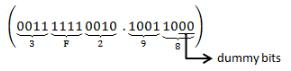(3F2.98)16
 Question 73
8-bit 1’s complement form of –77.25 is
 A 1.0011e+06 B 1.0011e+06 C 1.011e+07 D 1.011e+07
Digital-Logic-Design       Number-Systems       UGC NET CS 2011 June-Paper-2
Question 73 Explanation:
(-77.25)10 Step 1: Convert (77)10into binary form.Question 74
In which of the following codes the successive numbers differ in only one bit position ?
 A ASCII B Gray Code C Excess-3 Code D BCD
Digital-Logic-Design       Number-Systems       NIELIT Technical Assistant_2016_march
 Question 75
The binary addition of 1+1+1 is
 A 111 B 10 C 110 D 11
Digital-Logic-Design       Number-Systems       NIELIT Technical Assistant_2016_march
 Question 76
Which one of the following is decimal value of a signed binary number 1101010, if it is in 2’s complement form ?
 A -42 B -22 C -21 D -106
Digital-Logic-Design       Number-Systems       UGC NET CS 2013 June-paper-2
Question 76 Explanation:
Step-1: 2's complement number are weighted number. So, 1101010= -1*(0.26) + 1*25 + 1*23 + 1*21 = -64 + 32 +8 +2 = -22 Hence (1101010)=(-22) in 2's complement form
 Question 77
Which of the following weights makes the complement operation easier in BCD form ?
 A 8-4-2-1 B Excess-3 C 2-4-2-1 D 3-2-1-0
Digital-Logic-Design       Number-Systems       NIELIT Technical Assistant_2016_march
 Question 78
Let a n a n−1 ...a 1 a 0 be the binary representation of an integer b . The integer b is divisible by 3 if
 A the number of one’s is divisible by 3 B the number of one’s is divisible by 3, but not by 9 C the number of zeroes is divisible by 3 D the difference of alternate sum, i.e., ( a 0 + a 2 + . ..) − ( a 1 + a 2 + . ..) is divisible by 3
Digital-Logic-Design       Number-Systems       NIELIT Technical Assistant_2016_march
 Question 79
The decimal number equivalent of (4057.06)8 is
 A 2095.75 B 2095.07 C 2095.94 D 2095.09
Digital-Logic-Design       Number-Systems       UGC NET CS 2010 Dec-Paper-2
Question 79 Explanation:Question 80
12-bit 2’s complement of –73.75 is
 A 1.001e+06 B 1.1001e+07 C 1.01101e+07 D 1.01101e+07
Digital-Logic-Design       Number-Systems       UGC NET CS 2010 Dec-Paper-2
Question 80 Explanation:Question 81
Encoding of data bits 0011 into 7-bit even Parity Hamming Code is
 A 0011110 B 0101110 C 0010110 D 0011100
Digital-Logic-Design       Number-Systems       UGC NET CS 2010 Dec-Paper-2
Question 81 Explanation:
(m) Data = 0011
No. of parity bits needed is decided using
2p≥ m+p+1
p=3
Bit pattern: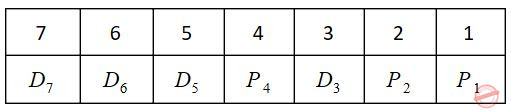P1(check even parity at 1, 3, 5, 7 bit) = 0
P2(check even parity at 2, 3, 6, 7 bit) = 1
P4(check even parity at 4, 5, 6, 7 bit) = 1
So encoded data = 0011110
 Question 82
What is decimal equivalent of BCD 11011.1100 ?
 A 22.0 B 22.2 C 20.2 D 21.2 E None of the above
Digital-Logic-Design       Number-Systems       UGC NET CS 2010 June-Paper-2
Question 82 Explanation:
The question is not properly framed.
In the question it is mentioned that 11011.1100 is BCD.
We know that BCD code is different from Binary number system.
Each of the decimal digit has a 4-bit binary code or in other words every block of 4 bits has a corresponding decimal digit.
0000- 0
0001- 1
:
:
1001- 9
1010 - 1111 are not valid codes.
If the given code 11011.1100 is BCD then divide it into blocks of 4 bits.
0001 1011. 1100 (Note: Appending zeros on the left side will not change value.)
But 1011 is not a valid BCD code.
 Question 83
In order that a code is ‘t’ error correcting, the minimum Hamming distance should be :
 A t B 2t-1 C 2t D 2t+1
Digital-Logic-Design       Number-Systems       UGC NET CS 2009-June-Paper-2
Question 83 Explanation:Question 84
The octal equivalent of hexadecimal (A.B)16 is:
 A 47.21 B 12.74 C 12.71 D 17.21 E None of the above
Digital-Logic-Design       Number-Systems       UGC NET CS 2009-June-Paper-2
Question 84 Explanation: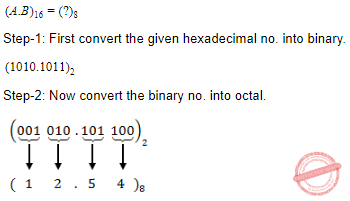Question 85
The answer of the operation (10111)2* (1110)2 in hex equivalence is
 A 150 B 241 C 142 D 101011110
Digital-Logic-Design       Number-Systems       UGC NET CS 2009 Dec-Paper-2
Question 85 Explanation:Question 86
How many 1’s are present in the binary representation of 3 × 512 + 7 × 64 + 5 × 8 + 3
 A 8 B 9 C 10 D 11
Digital-Logic-Design       Number-Systems       UGC NET CS 2009 Dec-Paper-2
Question 86 Explanation:
Given expression is 3 × 512 + 7 × 64 + 5 × 8 + 3
We can write above statement based on precedence is (3 * 512) + (7 * 64) + (5 * 8) + 3
Step-1: 1536+448+40+3
Step-2: (2027)10
Step-3: Equivalent binary number is (‭011111101011‬)2
Note: Total number of 1’s is 9.
 Question 87
If a code is ‘t’ error detecting, the minimum hamming distance should be equal to :
 A t-1 B t C t+1 D 2t+1
Digital-Logic-Design       Number-Systems       UGC NET CS 2008 Dec-Paper-2
Question 87 Explanation: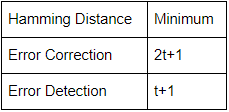Question 88
If a code is t-error correcting, the minimum Hamming distance is equal to :
 A 2t+1 B 2t C 2t-1 D t-1
Digital-Logic-Design       Number-Systems       UGC NET CS 2008-june-Paper-2
Question 88 Explanation:Question 89
The octal equivalent of the hexadecimal number FF is :
 A 100 B 150 C 377 D 737
Digital-Logic-Design       Number-Systems       UGC NET CS 2008-june-Paper-2
Question 89 Explanation:
Step-1: Convert FF into binary number
(FF)16 = (1111 1111)2
Step-2: Divide binary number into 3 segments from LSB(Least significant bit).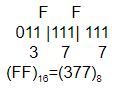Question 90
2’s complement of -100 is :
 A 00011100 B 10011101 C 10011100 D 11100100
Digital-Logic-Design       Number-Systems       UGC NET CS 2007 June-Paper-2
Question 90 Explanation:Question 91
How many 1’s are present in the binary representation of 15*256 + 5*16 + 3 :
 A 8 B 9 C 10 D 11
Digital-Logic-Design       Number-Systems       UGC NET CS 2007 June-Paper-2
Question 91 Explanation:
Step-1: The precedence to given decimal number is (15*256) + (5*16) + 3
Step-2: 3840+80+3=(3923)10
Step-3: Convert decimal number into binary
(3923)10 = (‭111101010011‬ )2
Step-4: Total number of 1’s are 8.
 Question 92
Let C be a binary linear code with minimum distance 2t + 1 then it can correct upto _____ bits of error.
 A t + 1 B t C t - 2 D t / 2
Digital-Logic-Design       Number-Systems       UGC NET CS 2017 Jan- paper-3
Question 92 Explanation:
A binary linear code with minimum distance 2t + 1 then it can correct up to ‘T’ bits of error.
There are 92 questions to complete.